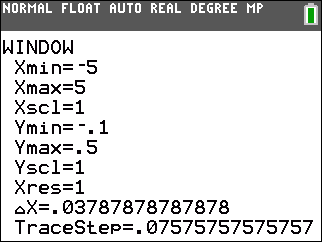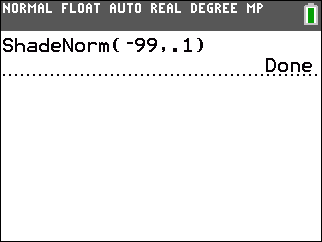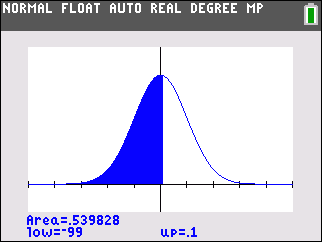# Knowledge Base

## Solution 34508: Computing the Z-Score on a TI-84 Plus C Silver Edition.

### How do I compute the Z-score on TI-84 Plus C Silver Edition?

A Z-score is a value obtained when data from a symmetric distribution is standardized. The formula for determining the Z-score is:

z= (x-m)/s

Where x is the observation,  m is the mean and  s is standard deviation.

A standard observation is used to determine how many standard deviations the original observation falls from the mean. Z-scores can be looked up in a table (z-table) to find the area under the standard normal curve (or probability) to the left of the z-value.

On TI-84 Plus C Silver Edition, the area under the standard normal curve for a z-value of .1 can be found by entering the following:

• Set your window settings, by pressing [WINDOW] and setting your values to xmin = - 5, xmax = 5, ymin = -.1 and ymax = .5.• Press [2nd] [CATALOG] [S] and locate the ShadeNorm( command and press [ENTER].
• Press [(-)]   [,] [.]  [)].• Press [ENTER].

The probability associated with a Z-score of .1 is shown in the bottom-left corner.Please see the TI-84 Plus C Silver Edition guidebook for additional information.